§ 5    Preliminary Riemannian geometry

1. Riemann space

[ Riemann space and its metric tensor ]  If there is a set of functions g ij ( x i )= g ji ( x i ) in the n -dimensional space R n , such that two adjacent points x i ,

The distance ds between x i + d x i is given by a positive definite quadratic form

d s 2 = g ij ( x )d x i d x j

Determined, then the space R n is called Riemann space, denoted as V n . The geometry in the Riemann space V n is called Riemann geometry . The quadratic type ds 2 is called the line element of V n . Differentiate into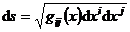And the arc length of any curve xi =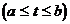xi ( t ) is the integral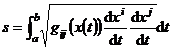because of the coordinate transformation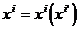Next, ds 2 is an invariant, so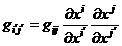This shows that g ij ( x ) is a component of a second-order covariance tensor, which is called the metric tensor or fundamental tensor of the Riemann space V n .

[ Length of vector, scalar product and angle of two vectors, adjoint tensor ] The definitions    of scalar ( field ) , vector ( field ) , tensor ( field ) , etc. in Riemann space are similar to the previous sections, and their operations The rules are also similar .

Let it be a contravariant vector, then the square of its length is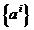g ij a i a j

If and are two contravariant vectors, their scalar product is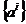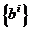g ij a i b j

The cosine of the angle between the two vectors is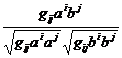Assume

g ij a i = a j , g ij b i = b j

Then and are covariant vectors, their length and scalar product are respectively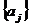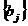g ij a i a j = a j a j , g ij a i b j = a j b j

The adjoint tensor of the tensor is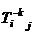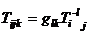,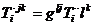where g lj satisfies the equation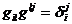where is the Kronecker notation .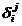[ Riemann contact and Christopher symbol ]  In Riemann space, the contact can always be determined in a unique way , satisfying the conditions: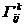(i)   Affine connections are torsion-free, i.e.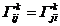(ii) The parallel movement generated by the affine connection keeps the length of the vector constant .

This is called the Riemann connection or the Levy-Civita connection .According to the above two conditions, it can be obtained that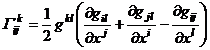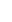If you remember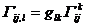then there are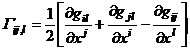Sometimes the following notation is used: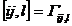and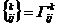They are called Christopher's three-index symbols of the first and second types, respectively .

In addition, there is the equation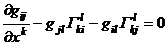or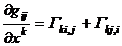It should also be pointed out that all the results in § 4 concerning the covariant differential method hold for the Riemann connection .2. The parallelism of Levi-Civita

The parallel movement in the affine connection space is determined by the affine connection . In the Riemann space V n with the metric tensor g ij , the Riemann connection is used to define the corresponding parallel movement called the Levy- Civita parallel movement .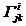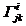Suppose a vector field a i = a i ( t ) is given along a certain curve x i = x i ( t ) in V n , if an infinitesimal displacement is made along this curve, the vector a i ( t ) follows the law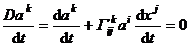change, then the vector a i ( t ) is said to perform a Levi-Civita parallel translation along the curve .

The Levi-Civita parallel motion has the properties:

1 The covariant derivative of the metric tensor g ij is equal to zero, i.e.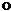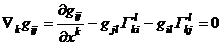Also ,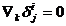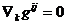2If the two families of vectors a i ( t ) and b i ( t ) move parallel to the curve, then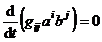Therefore, the scalar product of the two vectors and the included angle remain unchanged under parallel translation .

3The self-parallel curves ( also called geodesics ) in the Riemann space V n are exactly the same as the self-parallel curves in the affine connection space, both of which are determined by the differential equation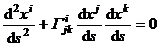But here is the Riemann connection . So a necessary and sufficient condition for a curve to be a geodesic is that its unit tangent vectors are parallel to each other .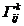3. Curvature in Riemann space

[ Curvature tensor and Lich's formula ]  The obvious difference between the covariant derivative of a tensor and the ordinary derivative is that when a higher-order derivative is obtained, the result of the tensor derivative is generally related to the order of the derivative . For example, when an operation acts on a vector , then there are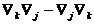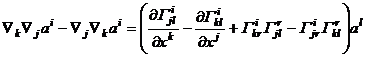(1)

remember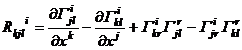It is a third-order covariant and first-order contravariant fourth-order mixed tensor, called the curvature tensor or Riemann-Christopher tensor of space V n .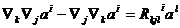The left side is called the staggered second-order covariant derivative of the contravariant vector ; the staggered second-order covariant derivative with respect to the covariant vector is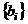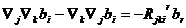The interleaved second-order covariant derivative of a tensor is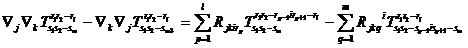This is called the Leach formula .

[ Riemann notation · Lich tensor · Curvature scalar · Einstein space ]

covariate component of curvature tensor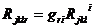are called Riemann symbols of the first kind, and Riemann symbols of the second kind .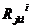The curvature tensor shrinks to get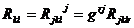is called the Ricci tensor . The Ricci tensor is then contracted to get

R = g kl R kl

called the curvature scalar .

If the Leach tensor satisfies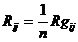This space is called Einstein space .

[ Properties of Curvature Tensors ]

1 The first two indices j and k of the curvature tensor are antisymmetric, i.e.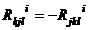very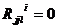2The curvature tensor performs cyclic permutation of the three covariant indicators and adds them, so that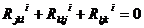This is called the Lich identity .

3The first kind of Riemann symbol R kjlr can be calculated as follows: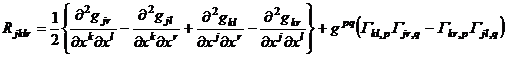Therefore , R kjlr is antisymmetric with respect to indexes j , k and l , r; it is symmetric with respect to the former pair of indexes and the latter pair of indexes; after cyclic permutation of the first three indexes, the sum is equal to zero, that is,

R jklr = - R kjlr

R jklr = -R jkrl _

R jklr = R lrjk

R jklr + R kljr + R ljkr = 0

4 The Lich tensor is symmetric, that is, R kl = R lk .At any point in the 5space V n , the following formula holds: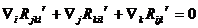This is called the Pianchi identity . It states that the sum obtained by cyclic permutation of the index ( i ) of the covariant derivative and the first two indices ( j , k ) of the curvature tensor is equal to zero .

[ Riemann curvature ( section curvature ) and constant curvature space ] do the  sum of two linearly independent vectors at a point M in the Riemann space V n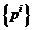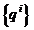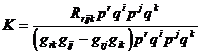This is called the Riemannian curvature of the plane determined by p i , q i , also known as the section curvature .

If for all points in space V n ( n > 2) we have

R rijk = K ( g rk g ijg rj g ik )

Then the Riemann curvature K is a constant, which is Schur 's theorem .

A space V n with a constant Riemann curvature is called a space of constant curvature, and the line elements of this space can be transformed into the form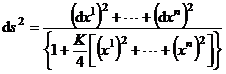This is called a measure of a space of constant curvature in Riemann form .

A space with constant curvature is an Einstein space .